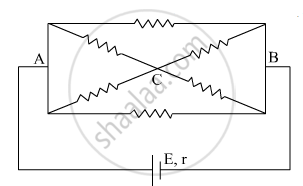Department of Pre-University Education, KarnatakaPUC Karnataka Science Class 12

# The current is drawn from a cell of emf E and internal resistance r connected to the network of resistors each of resistance r as shown in the figure. Obtain the expression for the current draw from the cell and the power consumed in the network. - Physics

The current is drawn from a cell of emf E and internal resistance r connected to the network of resistors each of resistance r as shown in the figure. Obtain the expression for

1. the current draw from the cell and
2. the power consumed in the network.#### SolutionFrom the above figure we can use the horizontal symmetry to deduce the current.

As both resistance in the circuit as well as the internal resistance in the circuit have the same resistance 'r'

The resistance of the loop I will be
1/R_I=1/r+1/(2r)

1/R_I=(3r)/(2r^2)

R_I=(2r)/3

Because the circuit I and II are same we can say
RII=(2r)/3
Combined resistance will be
R = RI + RII
1/R=1/R_(I)+1/R_(II)
1/R=1/((2r)/3)+1/((2r)/3)

1/R=2×(1/((2r)/3))

R=r/3
Now this circuit is series with internal resistance 'r'

Resultant resistance will be
R_("resultant")=(4r)/3

The current drawn from the cell will be
I=(3E)/(4r)

And power consumed by the network

P=I^2/R

P=(9E^2)/(16r^2)×(4r)/3

P=(3E^2)/(4r)

Concept: Kirchhoff’s Rules
Is there an error in this question or solution?